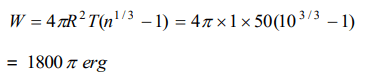## Mechanical Properties of Fluids Questions and Answers Part-6

1. Work done in splitting a drop of water of 1 mm radius into 106 droplets is (Surface tension of water = $72\times10^{-3} J\diagup m^{2}$    )
a) $9.58\times10^{-5} J$
b) $8.95\times10^{-5} J$
c) $5.89\times10^{-5} J$
d) $5.98\times10^{-6} J$

Explanation:2. A spherical liquid drop of radius R is divided into eight equal droplets. If surface tension is T, then the work done in this process will be
a) $2\pi R^{2} T$
b) $3\pi R^{2} T$
c) $4\pi R^{2} T$
d) $2\pi R T^{2}$

Explanation:3. If T is the surface tension of soap solution, the amount of work done in blowing a soap bubble from a diameter D to 2D is
a) $2\pi D^{2} T$
b) $4\pi D^{2} T$
c) $6\pi D^{2} T$
d) $8\pi D^{2} T$

Explanation: Work done to increase the diameter of bubble from d to D4. The radius of a soap bubble is increased from $\frac{1}{\sqrt{\pi}} cm$   to $\frac{2}{\sqrt{\pi}} cm$   . If the surface tension of water is 30 dynes per cm, then the work done will be
a) 180 ergs
b) 360 ergs
c) 720 ergs
d) 960 ergs

Explanation:5. If work W is done in blowing a bubble of radius R from a soap solution, then the work done in blowing a bubble of radius 2R from the same solution is
a) W/2
b) 2W
c) 4W
d) $2\frac{1}{3} W$

Explanation:6. A spherical drop of oil of radius 1 cm is broken into 1000 droplets of equal radii. If the surface tension of oil is 50 dynes/cm, the work done is
a) $18\pi$  ergs
b) $180\pi$  ergs
c) $1800\pi$  ergs
d) $8000\pi$  ergs

Explanation:7. The work done in blowing a soap bubble of radius r of the solution of surface tension T will be
a) $8\pi r^{2} T$
b) $2\pi r^{2} T$
c) $4\pi r^{2} T$
d) $\frac{4}{3}\pi r^{2} T$

Explanation: $8\pi r^{2} T$

8. If two identical mercury drops are combined to form a single drop, then its temperature will
a) Decrease
b) Increase
c) Remains the same
d) None of the above

Explanation: Surface energy of combined drop will be lowered, so excess surface energy will raise the temperature of the drop

9.If the surface tension of a liquid is T, the gain in surface energy for an increase in liquid surface by A is
a) $AT^{-1}$
b) $AT$
c) $A^{2}T$
d) $A^{2}T^{2}$

10. The surface tension of a soap solution is $2\times10^{-2}N\diagup m$    . To blow a bubble of radius 1 cm, the work done is
a) $4\pi\times10^{-6}J$
b) $8\pi\times10^{-6}J$
c) $12\pi\times10^{-6}J$
d) $16\pi\times10^{-6}J$